### Home > PC > Chapter 2 > Lesson 2.1.1 > Problem2-12

2-12.
1. Salvador prefers to evaluate 813/4 in a different way. He knows that 34 = 81, so he substitutes and uses the power law for exponents: 813/4 = (34)3/4 = 33 = 27. Use Salvador’s technique to evaluate each of these. Homework Help ✎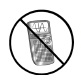1.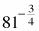2.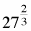3.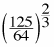4.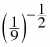Hint: Express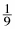as a power of 3.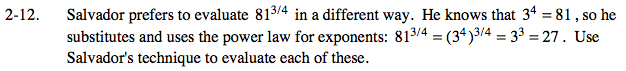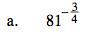$\frac{1}{27}$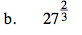9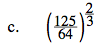$\frac{25}{16}$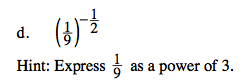3